# The cash cycle

• Study Notes
• Discuss This Topic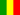Gabriel O. 1 0 The cash cycle has three distinct parts. The first part of the cycle represents the current inventory level and how long it will take the company to sell this inventory. This stage is calculated by using the days inventory outstanding calculation. The second stage of the cash cycle represents the current sales and the amount of time it takes to collect the cash from these sales. This is calculated by using the days sales outstanding calculation. The third stage represents the current outstanding payables. In other words, this represents how much a company owes its current vendors for inventory and goods purchases and when the company will have to pay off its vendors. This is calculated by using the days payables outstanding calculation.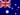Wendy C. 0 0 The cash cycle measures the time it takes to buy and sell stock, collect money from debtors and return that money to the business. Credit is also a factor.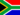Samuel F. 1 0 The cash conversion cycle is a cash flow calculation that attempts to measure the time it takes a company to convert its investment in inventory and other resource inputs into cash. In other words, the cash conversion cycle calculation measures how long cash is tied up in inventory before the inventory is sold and cash is collected from customers. The cash cycle has three distinct parts. The first part of the cycle represents the current inventory level and how long it will take the company to sell this inventory. This stage is calculated by using the days inventory outstanding calculation. The second stage of the cash cycle represents the current sales and the amount of time it takes to collect the cash from these sales. This is calculated by using the days sales outstanding calculation. The third stage represents the current outstanding payables. In other words, this represents how much a company owes its current vendors for inventory and goods purchases and when the company will have to pay off its vendors. This is calculated by using the days payables outstanding calculation.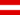Harrison A. 1 0 The cash cycle also known as cash flow can be analyzed over a long period of time,which can cover the various adverse phases,for determining the organisation's or firm's debt polity .The cash cycle analysis can be carried out by preparing a cash flow statement to show the firm's financial conditions under adverse condition such as recession . Cash cycle can be categorized into the following group or exampls of cash cycle are :Operating cash cycles ,None operating cash cycles , financial flows etc.Harrison A. 1 0 Give a distintive definition of cash flow or cycle .Zachary B. 0 0 What does the cash cycle include?Zachary B. 0 0 What is the cash cycle?Zachary B. 1 0 What does the cash cycle involve?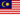Ella Rose M. 1 0 stock turnover + debt turnover - creditor turnoverDiamond T. 1 0 The cash conversion cycle is a cash flow calculation that attempts to measure the time it takes a company to convert its investment in inventory and other resource inputs into cash. In other words, the cash conversion cycle calculation measures how long cash is tied up in inventory before the inventory is sold and cash is collected from customers. The cash cycle has three distinct parts. The first part of the cycle represents the current inventory level and how long it will take the company to sell this inventory. This stage is calculated by using the days inventory outstanding calculation. The second stage of the cash cycle represents the current sales and the amount of time it takes to collect the cash from these sales. This is calculated by using the days sales outstanding calculation. The third stage represents the current outstanding payables. In other words, this represents how much a company owes its current vendors for inventory and goods purchases and when the company will have to pay off its vendors. This is calculated by using the days payables outstanding calculation.
• Text Version